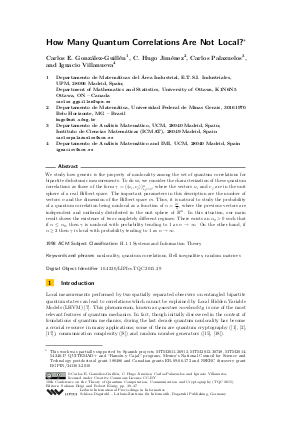Document# How Many Quantum Correlations Are Not Local?

### Authors Carlos E. González-Guillén, C. Hugo Jiménez, Carlos Palazuelos, Ignacio Villanueva## File

LIPIcs.TQC.2015.39.pdf
• Filesize: 445 kB
• 9 pages

## Cite As

Carlos E. González-Guillén, C. Hugo Jiménez, Carlos Palazuelos, and Ignacio Villanueva. How Many Quantum Correlations Are Not Local?. In 10th Conference on the Theory of Quantum Computation, Communication and Cryptography (TQC 2015). Leibniz International Proceedings in Informatics (LIPIcs), Volume 44, pp. 39-47, Schloss Dagstuhl - Leibniz-Zentrum für Informatik (2015)
https://doi.org/10.4230/LIPIcs.TQC.2015.39

## Abstract

We study how generic is the property of nonlocality among the set of quantum correlations for bipartite dichotomic measurements. To do so, we consider the characterization of these quantum correlations as those of the form gamma = ( < u_i , v_j > )_{i,j=1}^n , where the vectors u_i and v_j are in the unit sphere of a real Hilbert space. The important parameters in this description are the number of vectors n and the dimension of the Hilbert space m. Thus, it is natural to study the probability of a quantum correlation being nonlocal as a function of alpha = m/n , where the previous vectors are independent and uniformly distributed in the unit sphere of R^m. In this situation, our main result shows the existence of two completely different regimes: There exists an alpha_0 > 0 such that if alpha leq alpha_0, then gamma is nonlocal with probability tending to 1 as n rightarrow infty. On the other hand, if alpha geq 2 then gamma is local with probability tending to 1 as n rightarrow infty.
##### Keywords
• nonlocality
• quantum correlations
• Bell inequalities
• random matrices

## Metrics

• Access Statistics
• Total Accesses (updated on a weekly basis)
0